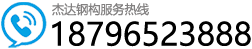MIC-240型拱型波纹屋盖计算书-相关知识-拱形波纹钢屋盖工程施工# MIC-240型拱型波纹屋盖计算书

### 一． 金属拱形波纹屋盖的材料选择

⒈ GB247 国家标准：钢板和钢带验收、包装、标志和质量证明书的一般规定.

⒉ 上海宝山钢铁厂企业标准：

① Q/BQB401-1999 冷连轧钢板和钢带的尺寸、外形、重量及允许偏差；

② Q/BQB440-1999 彩色涂层钢板和钢带；

3 BZJ441-1999 高耐候性冷连轧钢板和钢带。

### 三． 荷载及其组合

⑴ 恒荷载 包括屋盖结构自重、保温层和防火层自重、吊顶自重、采光带（窗）、通风帽等设备重量，灯具、管道等悬挂物重量；后两者可能是集中荷载，应尽可能沿屋盖跨度对称布置。材料自重标准值应按《建筑结构可靠性设计统一标准》GB 50068-2018 采用；各种设备和悬挂物重量按实际情况取值。

⑵ 竖向活荷载 包括积雪荷载、均布活荷载和积灰荷载，其中活荷载按不上人轻屋面考虑，取水平投影面屋面均布活荷载标准值为 0.5kN/m2，并不于雪荷载同时考虑，设计中时只取两者中的较大者；雪荷载和积灰荷载《建筑结构可靠性设计统一标准》GB 50068-2018 采用，积灰荷载应与活荷载或雪荷载中的较大者同时考虑。

⑶ 风荷载 屋盖在迎风的 1/4 弧面上受风压力，背风的 3/4 弧面上受风吸力。垂直于屋盖表面的风荷载标准值按照《建筑结构可靠性设计统一标准》GB 50068-2018 采用。

⑴ 恒荷载 + 活荷载 /雪荷载；

⑵ 恒荷载 + 风荷载；

⑶ 恒荷载+ 半跨雪荷载；

⑷ 恒荷载+ 风荷载+活荷载 /雪荷载；

⑸ 恒荷载 + 风荷载 + 半跨雪荷载（注意风向与半跨积雪的分布）；

⑹ 恒荷载+ 全跨非均布雪荷载（适用于连跨结构）；

⑺ 恒荷载 + 风荷载+ 全跨非均布雪荷载（适用于连跨结构）；

1.恒荷载 q恒荷载=q

(其中 7．85 为彩板质量密度，n 为单位投影面积重量系数=弧长/跨度*原始彩板宽度/成型后单元板宽度=15.9/15*914/610=1.588，t 为彩板厚度=1.2mm)

2.风荷载 q风=30kg/㎡

3.活荷载 q活=50kg/㎡

### 四． 结构单位宽度截面的各等效截面特征Aeq 等效截面面积=(8.5457+0.15614r-0.00223 r2 )/0.610=16.81cm2 /m

Ieq 等效截面惯性距=(342.88375+9.42483r－0.13479 r2 )/0.610=731.36cm4/m

W(1)eq 等效截面模量 1=(69.37162+0.59666r－0.00848r2)/0.610=75.91cm3/m

W(2)eq 等效截面模量 2=(25.78311+1.00009r－0.01393r2)/0.610=60.34cm3/m

Weq 等效截面模量=0.5*﹛W(1)eq+ W(2)eq﹜=49.15cm3/m

### 五． 计算各种荷载设计值下截面的组合弯距 Mi 和组合轴力 Ni

A. 在全跨竖向均布荷载作用下:

⑴ .. 支座的水平推力 H竖=A1*K* q 竖*L2/f=0.1235*1.0*0.94*152/2.25=11.61KN/m

【注:根据公式 H竖=A1*K* q 竖 L2/f 计算出支座的水平推力,A1(查建筑静力学计算手册表 6-5 得

kg/㎡=0.94KN/㎡,跨度 L=15m,矢高 f=2.25m,轴向力变形影响的修正系数 k≈1.0】

⑵.支座的垂直反力 R竖= q 竖*L/2=0.94*15/2=7.05KN/m

⑶.危险截面处的水平推力 H(D)竖=H竖=11.61KN/m

⑷.危险截面处的垂直反力 R(D)竖=R竖- q 竖* L(D)=7.05-0.94*2.81=4.4KN/m

⑸.危险截面处的轴向合力 N(D竖=H(D)竖* cos 260 +R(D)竖* sin 260=10.87KN/m

⑹.危险截面处的弯矩 M(D)竖= R竖* L(D) - H 竖 * f(D) - q 竖 * L(D) 2 /2=7.05*2.81-11.61*1.40-0.94*2.81 2 /2=-0.15KN.m/m

B. 在横向均布荷载作用下:

⑴. A 支座的水平推力 H横 a=-0.5*(1-A3)* q横 * f=-0.7140* 0.75*2.25=-1.20KN/m

⑵. B 支座的水平推力 H横 b=0.5*(1+A3)* q横 * f=0.2862* 0.75*2.25=0.48KN/m

【注:A3(查建筑静力学计算手册表 6-5 公式计算得)=-0.4281, q横=75kg/㎡=0.75KN/㎡】

⑶. 支座的垂直反力 R横=0

⑷. 危险截面处的水平推力 H(D)横 =H横 a – q(D)横* f(D)=-1.20-0.75*1.40=-2.25 KN/m

⑸. 危险截面处的垂直反力 R(D)横 = R横=0

⑹. 危险截面处的轴向合力 N(D横=H(D)横* cos 260 +R(D)横* sin 260=-1.46 KN/m

⑺. 危险截面处的弯矩 M(D)横= R横* L(D) – H 横 a * f(D) – q 横 * f(D) 2 /2=0.95KN.m/m

C. 危险截面处组合一阶轴力: N=N(D)竖 +N(D)

D. 危险截面处组合二阶弯矩:M= B1M(D)竖 + B2M(D)

qeri=KiEIi / r3=29.523*2.06*108*7.3*10-6/ 13.63=17.64 KN/m

B1=1/(1-riqi/ qeri)=1/(1-0.425*0.94/17.64)=0.98

M= B1M(D)竖 + B2M(D)

【注:弯矩放大系数 B,在风荷载作用下,取 B=1,即 B2=1;在其他荷载作用下按照公式 Bi=1/(1-riqi/qeri), 其中弹性临界荷载 qeri=KiEIi / r3 ;查规程 CECS167:2004 中表 B.0.1, C.0.1,得出:ri 弯矩调整系数=0.425, Ki 临界荷载系数=29.523; qi 荷载设计值=q竖=0.94KN/m,r 为拱形的曲率半径=13.6m,E为材料的弹性模量=2.06*108 KN/㎡, Ieq 等效截面惯性距=731.36cm4/m=4.4*10-6m4/m】

### 六． 承载力验算

N/A+|M/ Weq|=9.41/16.81*10-4 +0.80/ 49.15*10-6=21874KM/㎡=21.87N/mm2<f=235 N/mm2

H 风 A=1/2（1－A2）q 风 f（kN/m）（建筑结构静力计算手册 P305 表 6-5 中支座⑴HA 公式）

A2=－0.7140 见表）;q 风=0.75kN/ m ; f =2.25m

H 风 A=1/2（1+0.714）×0.75×2.25=1.46kN/m

H总（max）=（H竖）·a

a ——柱间距（5.4m）H 总（max）=（11.61）×5.4=62.69 kN

（注：可加钢绳预紧抵消：(H- 6 -总（max）-H 风 A*5.4)=54.81 kN

### 十二.伸缩缝设计### 欢迎 你 发表评论:

• 联系人：张凯（经理）
• 手机号码：18796523888
• 手机号码：18811000665
• 邮箱：79588724@qq.com
• 名称：江苏杰达钢结构工程有限公司
• 地址：江苏省盐城市建湖县经济开发区886号

• 文章总数:769
• 页面总数:1
• 分类总数:10
• 标签总数:185
• 评论总数:25
• 浏览总数:1336012